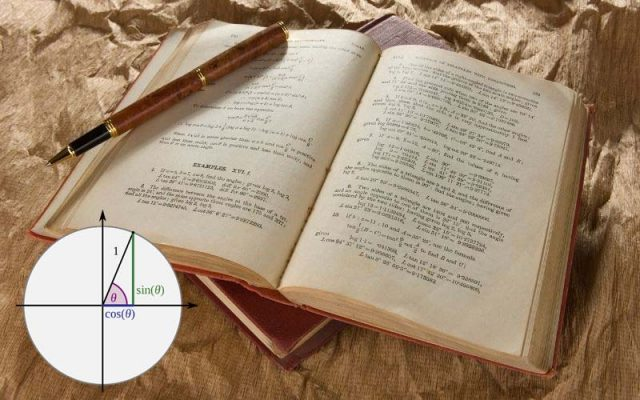# How were the Trigonometric Identities Created? Origin of TrigonometryTrigonometry is the branch of mathematics that aims to study the relationship between angles of triangles.

There are six functions of an angle, which are used commonly in trigonometry. Their names are sine, cosine, tangent, coscent, contingent second cause second.

The word trigonometry is derived from the Greek word trigonon which means triangle and metron which means to measure.

## History of TrigonometryThe history of trigonometry starts from the Hellenistic world in the 3rd century. It started when geometry was introduced to Astronomy.

The history of trigonometry starts from the Middle East and travels to Greeks, India, Arabic, and finally Europe.

## Greeks

Until exchange in theory it was chiefly concerned with computing numerical values of a triangle. For example, if plans of two are given the third side of the triangle it was calculated using trigonometry.

There are several Ancient civilizations in which trigonometry is seen.

The origin of trigonometry seen in Egyptian Babylonian Hindu and Chinese. They all possess knowledge of some practical geography.

The study of triangles can be traced back to the second century, second millennium BC.

## Ancient Egyptian and Babylonia

The ancient Egyptian and Babylonian had may have known theorems of the ratio of the size of similar triangles. However, they lack the concept of angle measure.

Therefore it limited their studying of sides of angles. However, their astronomers kept detailed records of the rising in the setting of stars, the motion of planets, and eclipses. Which were based on angular distance measured on the celestial sphere.

Egyptian on the other hand, used the earliest form of digital imagery for building pyramids in the second millennium BC.

The Rhind Mathematical Papyrus contains the following problems related to its, possibleator which is like this.

If a pyramid is to speak your words I divide it faces 360 cubits long What is it seked.

## Greeks

Trigonometry in the modern sense began with the Hellenistic world.

Hipparchus was the first person to construct a table of values for the trigonometric functions. He was an astronomer, therefore, was only interested in spherical triangles.

Till the 16th century trigonometry was used to measure the side of a trainable if two sides were given.

Another contribution to trigonometry was made After Dark Ages by Ptolemy. He lived in Alexandria which was an intellectual center of the Hellenistic world.

Nothing more is known about him. He wrote works on mathematics, geography, and others. But is chiefly known for his work Almagest

Almagest deals with the construction of a table of chords.

With the help of the Trigonometry table, he improved on existing geodetic measures of the world and refined the Hipparchus model of the motion of heavenly bodies.

His method of trigonometric calculation in astronomy was used for the next 1200 years.

## Contribution of India and the Islamic world

### The next contribution came from India

The modern sine convection was first assisted in Surya Siddhanta and its properties were first documented by Indian mathematics mathematician and astronomer Aryabhatta.

The first table of sines is found in Aryabhatiya.

### Arabic and Muslim World

Later this work reached Arabic by translation of translation by Muslim scholars.

Until the 10th century, Islamic mathematicians were using six trigonometric functions. Also, we are applying them to solve spherical geometry problems.

Nasir al-Din al-Tusi was the first to consider Trigonometry as a mathematical discipline and independent from astronomy. He wrote the Law of Sines for planes and spherical triangles.

Other Arabic scholars like Al Batani also made significant contributions in developing Trigonometry further.

### Modern Europe

This knowledge of function and method reached West Europe via Latin translation of Arabic works and also via translation of works of Ptolemy and others.

The mention of trigonometry De triangulos is first noted by Northern European mathematicians. Till the 16th-century Trigonometry wasn’t very important.

But in the 16th century with the rise in demand for navigation, Trigonometry became an unbroken part of mathematics.

Bartholomaeus Pitiscus was the first to use the word Trigonometry in his book in 1595.

Edmund Gunter was the first to use abbreviated symbols of Sine in 1624. He was an English minister and instrumental maker.

In the 16th century, trigonometry began to change its character from pure geometry to an algebraic analytic subject.

#### Conclusion

This is how Trigonometry developed. First used to calculate inclination, then used for astronomy.

Then finally changing its form into an algebraic analytical subject.

Moreover, the whole world made significant contributions to develop trigonometry in its modern form. From Greeks to India, from Arab to Europe.

I hope you would love this short history of trigonometry explained in the simplest word posible.